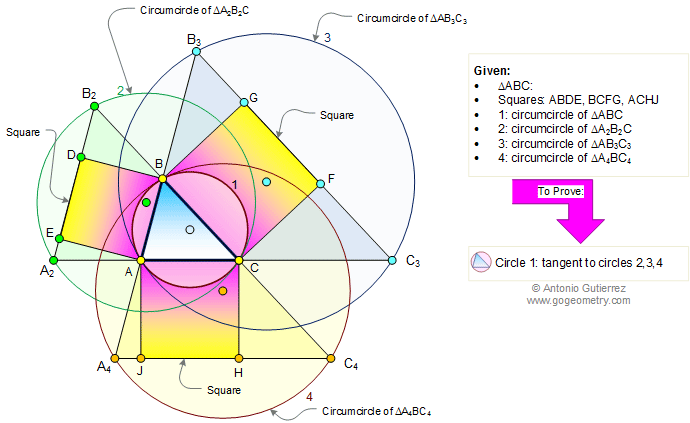Problem 370. Triangle with squares, Circumcircles, Tangent circles. Level: Math Education, High School, College, SAT Prep. The figure shows a triangle ABC with the squares ABDE, BCFG, and ACHJ. DE extended meet AC and BC extended at A2 and B2, respectively. GF extended meet AB and AC extended at A3 and C3, respectively. JH extended meet BA and BC extended at A4 and C4, respectively. Prove that the circumcircle of triangle ABC is tangent to circumcircles of triangles A2B2C, AB3C3, and A4BC4.Recent Additions